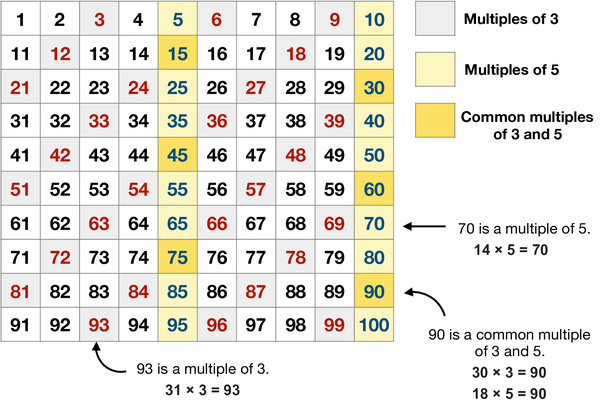# Multiples Shown on Hundreds ChartsAddition, subtraction, multiplication and division are the four elementary mathematical operations of arithmetic. The result of a multiplication operation is called a product. A multiple of a number is the product of this number and an integer. Multiples can be shown in arrays, on number lines, and on hundreds (100s) charts.

This worksheet provides a hundreds chart with multiples of 3, multiples of 5, and common multiples of 3 and 5 marked in different colors. With the help of this chart, parents could help kids learn, memorize and recall the multiplication of small numbers easily. It also includes two sheets of exercises. Parents could ask kids circle multiples of 3, 5, 7, 11 on 100s charts in the first exercise. When kids are more confident with multiplication, they can fill in multiples of 3, 5, 7, 11 on 100s charts in the second exercise.